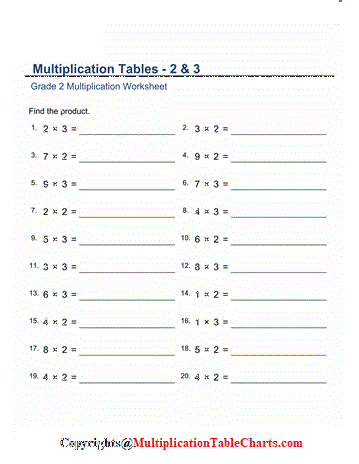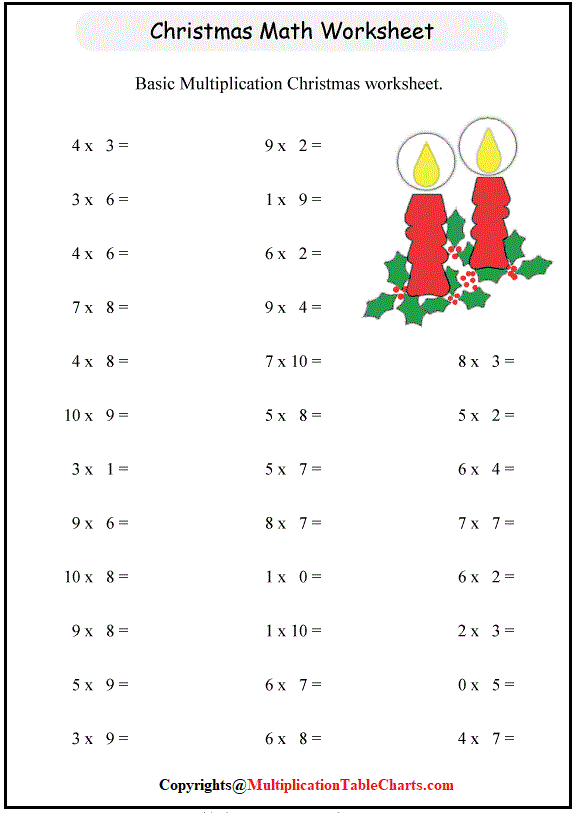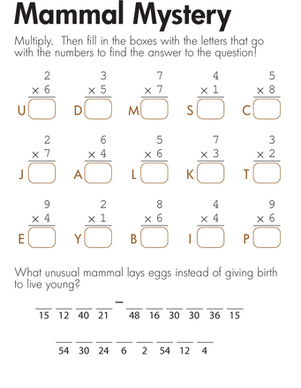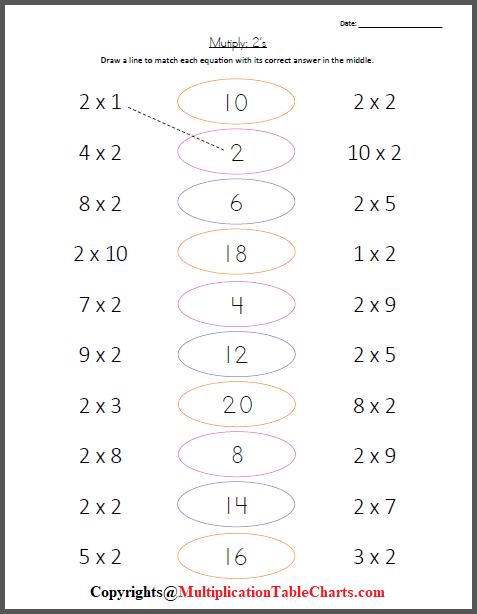# Free Printable Multiplication Worksheets For Grade 2 [PDF]

Multiplication Worksheets For Grade 2 is the repeated addition. This site provides you with the multiplication worksheet for grade 2. If you are a parent, this site will be very helpful to you as it provides a multiplication worksheet for grade 2. If you are a working mom it will be helpful as you can just take the printout of the worksheet from the site and give it to you as a little one for practice.

This worksheet will be very helpful for multiplication practice during math examinations. These worksheets present the meaning of multiplication with pictures; children choose the picture which matches the given multiplication sentence.

## Multiplication Worksheets For Grade 2

It is fun to learn numbers with pictures. Your kids are going to find it interesting to solve this attractive worksheet. Teachers with good experience have come up with these multiplication worksheets for grade 2. These grade 2 multiplication worksheets improve early multiplication skills; especially recall of the 2, 5 and 10 ‘times tables’, multiplying by whole tens and solving missing factor problem, also in these for grade 2, children first match the multiplication sentence to the picture, and then answer the multiplication sentence, using the picture as an aid, counting or skip counting as necessary.PDF

This worksheet also provides multiplication with arrays. Arrays can be used to understand multiplication in a better way.  The no. of rows of an array can represent the number of groups and the number of columns represents the number of objects per group.  The multiplication sentence is the number of rows times and the number of columns and the answer is easily found by skip counting.

### Multiplication Worksheets For Grade 2 PDF

Here you can get free printable multiplication worksheets for grade 2 in pdf format. If you have a printer at your home the printout will cost you nominal. The worksheet will have questions related to the table of 2, 3, 5 & 10 for example 2×5=__, 2×3=__, 2×10=__, etc. This worksheet will also have some questions related to skip counting. The missing factor questions like 2 x __ = 18, 2 x __ = 20 etc.PDF

This site will provide you with colorful and printable Multiplication worksheets for grade 2 pdf. You can just take out the print and give it to your child for practice. These multiplication worksheets will improve your child’s multiplication skills and also increase his/her interest in maths.

### Multiplication Worksheets For Grade 2 With Pictures

The Multiplication Worksheets For Grade 2 With Pictures will have word problems with pictures so that students may find it attractive. The multiplicands given here are single-digit numbers and all products are less than 20, so the computations are kept simple and the concepts understood.PDF

Here we also include irrelevant numbers in some problems, so that students must read and understand the question to answer it. These worksheets are ready to print in pdf format. The site has multiplication worksheets for grade 2 with pictures. The pictures are of high-resolution. The pictures make story/word problems attractive.

### Multiplication Word Problem Worksheets For Grade 2

It is so difficult for working parents to manage both work and kids. We cannot share the burden but for sure we can help a little through these Multiplication word problem worksheets for grade 2. It will also enhance your child’s multiplication skills.PDF

Here word problems are also given, all multiplicand is single digit and products are less than 20. The word problems are given strictly age-appropriate.  The multiplication word problem worksheets for grade 2 include all the simple questions and concepts that are simple to understand. Few examples of word problems are as follows:-

How many legs do 5 cats have?

A rocket firework explodes into 5 stars. How many stars will 7 rockets make?

I buy 5 packs of 5 biscuits each. How many biscuits in total?

Not only questions, but we also provide a guide showing the steps to solve these questions. We also give the answer key on the site. For example:-

As we know, multiplication is repeated addition; we can multiply 56 and 24 to get the answer.

56 ×24

• 224
• 1120
• 1344

[Sometimes, more than one calculation is required.]

Therefore, altogether there are 1344 sheets.

This multiplication word problem worksheets for grade 2 is a complete lesson for grade 2 with teaching & word problems to teach children some basics about multiplication word problems. Here we have groups of the same size, and children need to just recognize those groups, whether they are towels, pizza slices, balls, or whatever. The word problems in the lesson also involve addition and subtraction so that students need to think, and not apply the operation at hand (multiplication) without even reading the problem.

So grab the opportunity and download the free printable multiplication word problem worksheets for grade 2 in pdf format which will have high-resolution pictures.